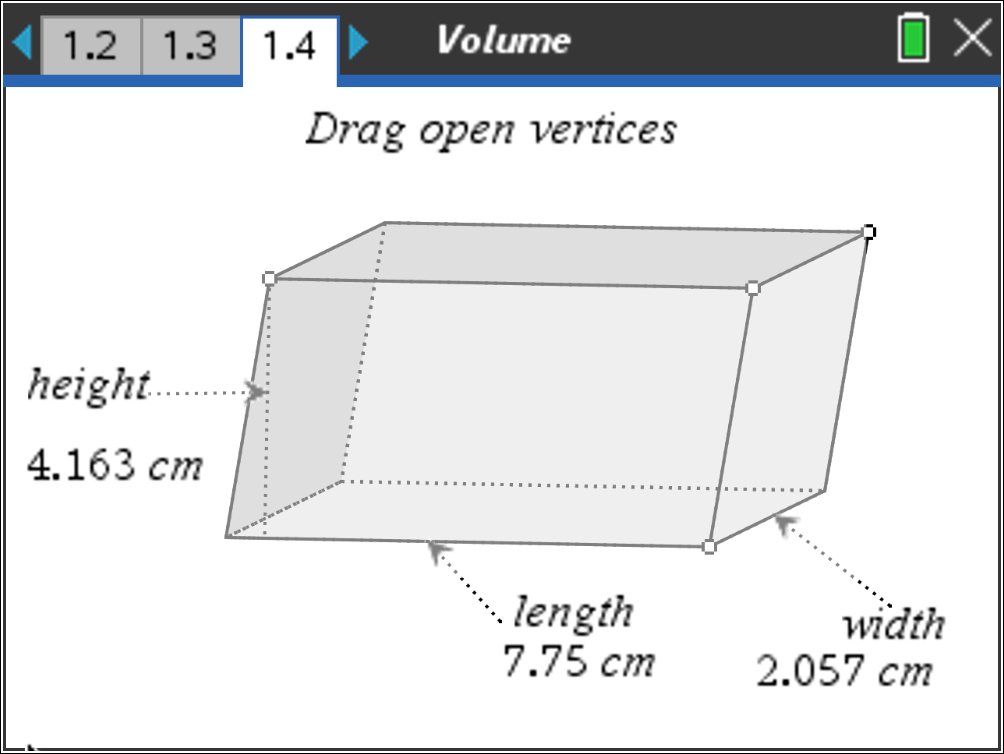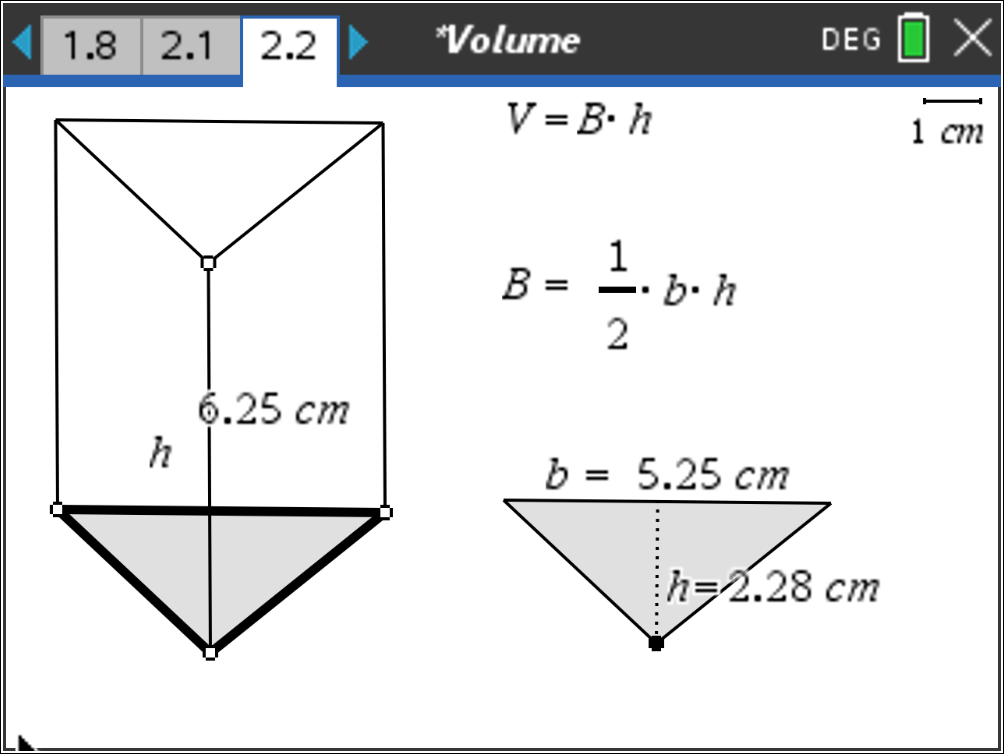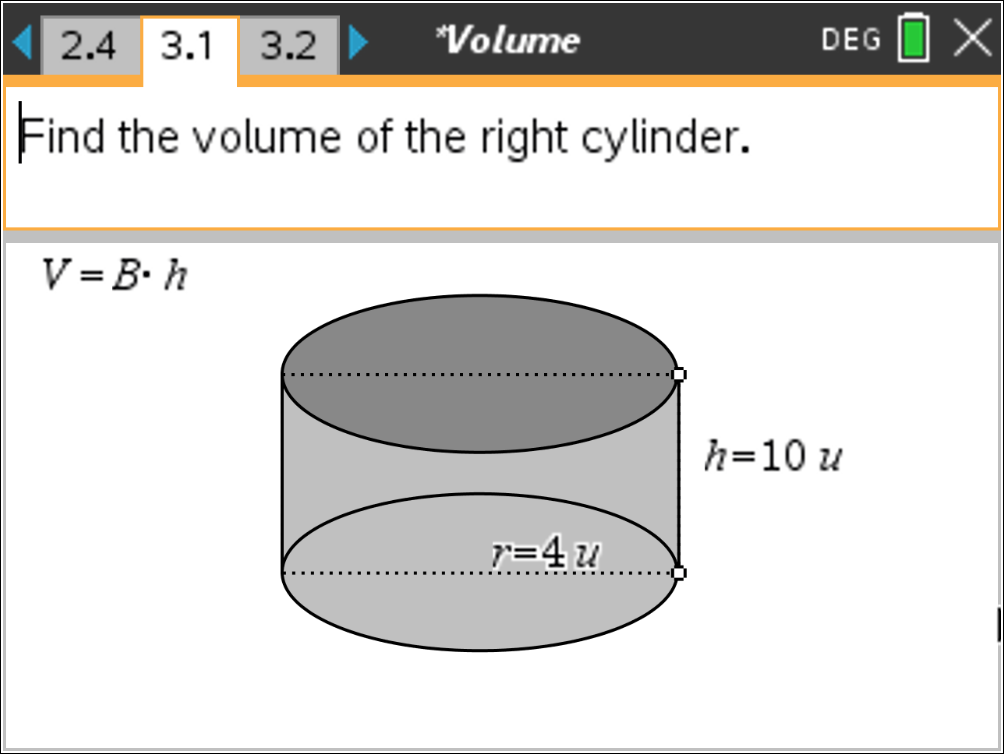### Mathematics lessons for IB® Diploma Programme

Applications and Interpretation | Geometry and Trigonometry## Volume

### Activity Overview

Students define right and oblique three-dimensional figures and calculate the volume for prisms, pyramids, cylinders and cones.

## Key Steps## Step 1

Students begin by exploring rectangular prisms. They use an interactive figure built into this activity, seen to the right. They drag the vertices of the prism and explore various shapes it takes on. Students must pay close attention to which vertex affects the length, width, and height of the prism.## Step 2

Problem 2 gives students the opportunity to explore triangular prisms and pyramids. Students use the given triangular prism to find its volume. They use the Calculate command and select the values needed to solve the area of a triangle B = ½• b • h; and the volume V = B • h.

In this problem, students will also discuss the difference between prisms and pyramids.## Step 3

Students move on to Problem 3 where they investigate the volume of a right cylinder and a cone. As before, students can drag the open points to change the height and radius of the base.